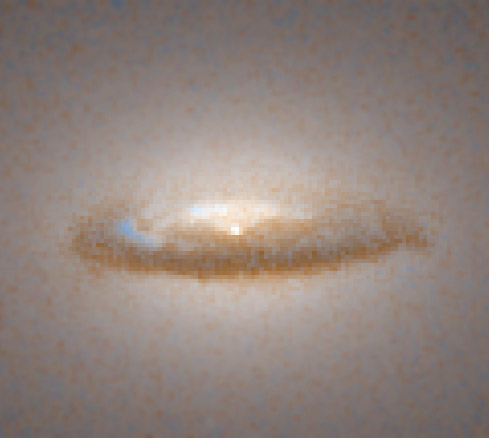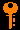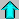# Lecture 19 - Black Holes (3/30/99)Pulsars --- | --- The GalaxyReading:
Chapter 17-3, 17-1E, Prelude P7-1 (ZG4)

Notes:
pages 76-81

Hubble Space Telescope image of the dust disk in the nucleus of the galaxy NGC7052, which is believed to harbor a 300 million solar-mass black hole! (Courtesy NASA/STSCI)
Key Question:## Investigations:

1. Spacetime and Special Relativity
• What is an inertial frame?
• What is an invariant interval in spacetime?
• Why is the speed of light a constant?
• How do you compute the length of spacetime vectors?
• What is relativistic time dilation?
• What is relativistic length contraction?
• What is the relativistic momentum and energy formulae?
• Why is the notion of simultaneity inappropriate in relativity?
2. General Relativity
• How can you tell if an elevator is under gravity or accelerating from inside?
• What is a freely falling frame?
• How can light travel on straight lines when it is clearly bent by gravity?
• How is spacetime curved by the presence of mass?
• What is a geodesic?
• What is meant by non-Euclidean geometry?
3. Black Holes and Relativity
• What is the event horizon?
• How does the black hole horizon area scale with mass?
• Can the radius of a black hole decrease?
• What three macroscopic quantities characterize a black hole?
4. Brown Dwarfs
• What is the smallest mass main sequence spectral type?
• What is a brown dwarf?
• What differentiates a star from a gas giant planet?
• What does the spectrum of a brown dwarf look like?

## Black Holes in the UniverseThe center of active galaxy Centaurus-A, which likely contains a supermassive black hole. Courtesy HST/STSCI

There are a number of spectacular images of systems believed to harbour stellar-mass and supermassive black holes. The Hubble Space Telescope in particular has played an important role in pinning down these elusive objects. Some examples:

## Black Hole Effects:

The angle of gravitational deflection of light rays passing the limb of an object:

 Sun 1".75 White Dwarf 1' Neutron Star 30o Black Hole ???

The presence of a strong gravitational field, like near the surface of a compact object like a white dwarf, neutron star, and black hole causes the wavelength of light to increase, just like the Doppler effect for a source moving away from the observer. Light coming from a source at the event horizon of the black hole to an observer far from the black hole is redshifted to infinite wavelength.

Because gravity causes the warping of space and time, a light ray passing near a compact object is time-delayed. That is, it takes longer for the photon to travel a path passing near a large mass, than it does for the same path were the mass not there. When we discuss general relativity below, we will see that this is not because light is slowed (the speed of light is a constant in vacuum) but because space-time is curved. A light ray passing the limb of a black hole (the event horizon) is infinitely delayed, though not completely captured.

These strong forces, along with the tremendous gravitational energy gained by matter falling from a large distance, cause gas swept up by the black hole to form an accretion disk. Like the accretion disk of a neutron star, this gas would be heated to extemely high temperatures and would emit large numbers of X-rays and Gamma Rays from the thermal emission alone.

## Black Hole Lore:

Black holes do not suck up matter like a cosmic vacuum cleaner. The gravitational force at a given distance from a black hole is the same as any spherical body of the same mass. It is just that you can get so much closer relative to where the mass is concentrated.

What enters a black hole event horizon appears to be lost from the outside world forever. Does that mean that information sent into the black hole (like matter, quantum numbers, etc.) are lost forever?

## Special Relativity:

1. The postulate of relativity: The laws of physics are the same for all inertial (uniformly moving) observers.
2. The constancy of the speed of light: The speed of light is measured to be the same for all inertial observers, regardless of their motion relative to the source of the radiation. (This was new, and the crucial new ingredient.)

The relativity postulate is the same as Galileo's. These uniformly moving frames of reference are called inertial or "Galilean" because Galileo's law of inertia holds in them.

The postulate of the constancy of the speed of light for all observers turns out to be deep. Suppose I turn on a flashlight while flying my spaceship past you at 0.5c. You might suppose that the speed of the photons will be 1.5c, but this is not the case, the photons move at c. Furthermore, we both measure the velocity of the photons to be the same: 3x10^5 km/s!

See the Special Lecture on the speed of light for more details.

If the speed of light is the same for all observers, then some thing must be different to compensate for the different relative velocities. It turns out that our measurements of lengths and time intervals that change.

To understand this, we must think not of three dimensional space, and time, as separate entities, but of a four-dimensional spacetime.

The relativistic effects on the length, time, mass, wavelength get extreme as the velocity approaches the speed of light. The common factor in all these is the Lorentz factor (this is supposed to be the greek letter gamma):

y = [ 1 - v2/c2 ]-1/2

As v approaches c, then gamma approaches infinity as the denominator goes to zero

v/c y
0 1
0.1 1.005
0.5 1.155
0.9 2.294
0.99 7.089
0.999 22.37
0.9999 70.71

## General Relativity:

Einstein's General Theory of Relativity (1916) deals with the laws of physics for accelerated observers, just as the Special Theory did for uniformly moving observers. The cornerstone of this theory is the equivalence principle: the laws of physics are the same for all freely falling observers. A freely falling observer is an accelerated observer who is in motion as defined by a gravitational field (without fighting against it). A spaceship or planet in orbit is freely falling (though not falling in the colloquial sense), as is a plummeting airplane. Someone standing on the ground is not, because the ground is exerting a counter-force to the gravitational force on us.

There is no gravitational force on an body infinitely far from all masses in the universe. This body would be un-accelerated, and would remain in uniform motion forever, and would be in all senses "weightless" (though not massless or inertia-less). We know from experience (or at least watching astronauts experience it) that things in a spaceship orbiting the Earth appear also to be "weightless". However, the fact that they are in orbit shows that there is gravitational force (not too much less than on the Earth's surface since an orbit at 500km is only a fraction more than at the Earth's radius of 6400km). It is just that everything in the shuttle feels the same acceleration and is accelerated together. Likewise, a diving airplane (or falling elevator) can provide the experience of weightlessness even in the presence of gravity - this is how the film Apollo 13 was shot.

What Einstein realised is that this was not just an illusion, but that it was a deep principle (like the constancy of the speed of light). He postulated that the laws of physics were the same for all freely falling observers, and furthermore an accelerating observer is equivalent to being in a gravitational field --- there is no difference! Artificial gravity from an accelerating rocket (or an accelerating elevator, car, or jet) is equivalent to real gravity!

Light (a photon) is always freely falling. It should always follow straight line path even in the presence of gravity. But light is deflected by gravity, and appears to follow a curved path. Therefore, it must be that spacetime is curved in the presence of mass - this is the manifestation of gravity. Gravity is the curvature of spacetime in the presence of mass.

In (possibly curved) spacetime, light follows geodesics (straight lines). An analog is drawing a straight line on a sphere, like the surface of the Earth. We can draw a straight line, like a long long road, but eventually we will find our selves back where we started! A straight line on the curved surface of the sphere is a great circle (like the equator, or any meridian of longitude). But is, as far as someone confined to the surface is concerned, straight. This is the same sort of curvature that mass can have on spacetime.Prev Lecture ---Next Lecture ---Astr12 Index ---Astr12 Home

smyers@nrao.edu Steven T. Myers